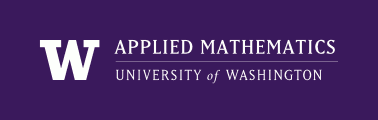AMath 584, Autumn Quarter, 2011 Applied Linear Algebra and Introductory Numerical Analysis

Syllabus¶

We will cover much of parts I through V of Trefethen and Bau, along with some supplementary material.

Some major topics¶

• Review of basic linear algebra in finite dimensional spaces, including both R^n and also function spaces.
• Linear independence, bases, norms, matrix factorization, etc.
• Orthogonality and the Singular Value Decomposition (SVD).
• Least squares problems: QR factorizations, Gram-Schmidt, Householder transformations.
• Conditioning of problems and stability of algorithms.
• Linear systems of equations: Gaussian elimination and LU factorizations.
• The eigenvalue problem: the power method and QR algorithms, relation to SVD.
• Various applications of the above algorithms will also be considered.